﻿ An Application of Ergodic Markov Chain to the Process of Teaching MathematicsPublications are Open
Access in this journal
Article Versions
Export Article
• Normal Style
• MLA Style
• APA Style
• Chicago Style
Research Article
Open Access Peer-reviewed

### An Application of Ergodic Markov Chain to the Process of Teaching Mathematics

Michael Gr. Voskoglou
American Journal of Applied Mathematics and Statistics. 2019, 7(5), 187-190. DOI: 10.12691/ajams-7-5-5
Received September 19, 2019; Revised October 27, 2019; Accepted November 18, 2019

### Abstract

The principles of social constructivism for learning have become very popular in the recent decades for teaching mathematics. In the present paper a mathematical representation is created of the process of teaching mathematics (based on those principles) by applying an ergodic Markov chain on its steps. This enables the instructor to evaluate the student difficulties in the classroom and therefore to reorganize his (her) plans for teaching the same subject in future. A classroom application to teaching the Conic Sections to engineering students is also presented illustrating the usefulness of our Markov chain model in practice.

### 1. Introduction

The process of learning is fundamental to the study of the human cognitive action. There are many theories and models designed for the description of the mechanisms of learning. Volumes of research have been written about it and many attempts have been made by psychologists, cognitive scientists and educators to make learning accessible to all in various ways.

There are four main philosophical frameworks under which learning theories fall. These are: Behaviorism, Cognitivism, Constructivism and the Socio-cultural approach. Behaviorism focuses only on the objectively observable aspects of learning; for behaviorists learning is the acquisition of new behavior through conditioning. Cognitive theories look beyond behavior to explain brain-based learning, while constructivism views learning as a process in which the learner actively constructs or builds new ideas and concepts. On the other hand, the socio-cultural views for learning are based on the Vygotsky’s ideas 1 that knowledge is a product of culture and social interaction. Learning takes place when the individuals engage socially to talk and act about shared problems or interests 2. The combination of constructivism with the socio-cultural ideas is known as social constructivism 3.

Mathematics teaching is intended to promote the learning of mathematics. But, while theories enable us to analyze and explain the process of learning, they rarely provide direct guidance or practical rules for promoting the teaching process.

The application of the principles of social constructivism have become very popular for teaching mathematics since the 1980’s, when the failure of the introduction of the “new mathematics” to school curricula had been already evident to everybody ( 4, Section 6). The steps of a typical teaching framework based on those principles could be considered to be the following:

Ÿ Orientation (S1): This is the starting step which connects the past with the present learning experiences and focuses student thinking on the learning outcomes of the current activities.

Ÿ Exploration (S2): In this step students explore their environment to create a common base of experiences by identifying and developing concepts, processes and skills.

Ÿ Formalization (S3): In this step students explain and verbalize the concepts that they have been explored and they develop new skills and behaviors. The instructor has the opportunity to introduce formal terms, definitions and explanations for the new concepts and processes and to demonstrate new skills or behaviors.

Ÿ Assimilation (S4): Here students develop a deeper and broader conceptual understanding and obtain more information about areas of interest by practicing on their new skills and behaviors.

Ÿ Assessment (S5): This is the final step of the teaching process, where learners are encouraged to assess their understanding and abilities and teachers evaluate student skills on the new knowledge.

Depending on the student reactions in the classroom, there are forward or backward transitions between the three intermediate steps (S2, S3, S4) of the above framework during the teaching process.

It is of worth noticing that those three steps are actually the Polya’s consecutive phases for teaching mathematics with the method of discovery 5, which is highly based on active learning. The last one is an idea adopted from Piaget, who is considered to be the forerunner of the constructivism, although the corresponding theory was formally introduced by von Clasersfeld 6.

The consecutive phases and the active learning are the first two of the Polya’s three famous axioms of learning 7, for which he used to say that they are not of his own conception, but they have been derived through the experience of centuries. The third axiom is the best motivation (a problem, a real life situation, a story, etc.), which is connected to the step of orientation (S1) and has to do with the suitable “learning situation” that has to be created by the instructor connecting the previous to the new knowledge and increasing the student interest about it.

The objective of the paper at hands is to create a mathematical representation of the process of teaching mathematics by applying an ergodic Markov chain on the steps of the previous teaching framework. The rest of the paper is formulated as follows: In Section 2 the necessary for our purposes information from the theory of ergodic Markov chains is presented. In Section 3 the Markov chain model is developed and through it some measures are obtained for evaluating the student difficulties in the classroom during the teaching process. An application of the model is presented in Section 4 and the paper closes with the conclusions and some hints for future research that are stated in Section 5.

### 2. Ergodic Markov Chains

It is recalled that a Markov chain (MC) is a stochastic process that moves in a sequence of steps (phases) through a set of states and has a one-step memory. In other words, the probability of entering a certain state in a certain step depends on the state occupied in the previous step and not in earlier steps. This is known as the Markov property. However, for being able to model as many real life situations as possible by using MCs, one could accept in practice that the probability of entering a certain state in a certain step, although it may not be completely independent of previous steps, it mainly depends on the state occupied in the previous step 8.

When the set of states of a MC is a finite set, then we speak about a finite MC. Let us consider a finite MC with n states, say S1, S2, …, Sn, where n is a non negative integer, n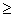2. Denote by pij the transition probability from state Si to state Sj , i, j = 1, 2,…, n ; then the matrix A= [pij] is called the transition matrix of the MC. Since the transition from a state to anyone of the other states (including its self) is the certain event, we have that(1)

The row-matrix Pk = [p1(k) p2(k)… pn(k)], known as the probability vector of the MC, gives the probabilities pi(k) for the MC to be in state i at step k , for i = 1, 2,…., n and k = 0, 1, 2,…. Obviously we have again that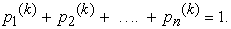(2)

It is well known ( 9, Chapter 2, Proposition 1) that Pk+1= Pk A (3), for all non negative integers k. Therefore a straightforward induction on k gives that(3)

for all integers k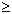1.

Formula (4) enables one to make short run forecasts for the evolution of the various situations that can be represented by a finite MC.

In practical applications we usually distinguish between two types of finite MCs, the absorbing and the ergodic ones. In recent works we had the opportunity to apply the theory of the absorbing MCs to problem-solving 10 and to the Bloom’s taxonomy for learning 11. Here we are going to apply the theory of ergodic MCs for representing the process of teaching mathematics.

A MC is said to be an ergodic MC (EMC), if it is possible to go between any two states, not necessarily in one step. It is well known ( 12, Theorem 5.1.1) that, as the number of its steps tends to infinity (long run), an EMC tends to an equilibrium situation, in which the probability vector Pk takes a constant price P = [p1 p2 …. pn], called the limiting probability vector of the EMC.

Therefore, as a direct consequence of equation (3), the equilibrium situation is characterized by the equation(4)

with p1+ p2+ ….+ pn = 1.

The entries of P express the probabilities of the EMC to be in each of its states in the long run, or in other words the importance (gravity) of each state of the EMC.

Let us now demote with mij the mean number of times in state Si between to successive occurrences of the state Sj, i, j = 1, 2, …., n. It is well known then that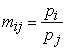(5)

where Pi and Pj are the corresponding limiting probabilities ( 12, Theorem 6.2.3).

### 3. The EMC Model

We introduce a finite MC with states the steps S1, S2, S3, S4 and S5 of the teaching framework described in our introduction. It is assumed that, when the teaching process of a certain mathematical topic is finished, then the instructor begins the teaching of a new topic. This means that the MC moves from state S5 back to its starting state S1 and the same process is repeated. Therefore, its flow-diagram is that shown in Figure 1.

View option
• Figure 1. The flow diagram of the MC

From Figure 1 it becomes evident that we have an EMC with transition matrix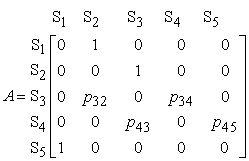with p32 + p34 = p43 + p45 = 1.

Therefore, equation (5) gives thatConsequently it turns out that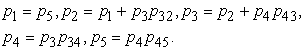(6)

Adding by members the first four of the equations (6) one finds that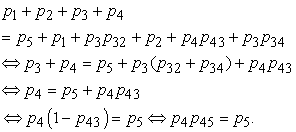Therefore, the fifth of the equations (6) is equivalent to the rest of them.

Consider now the linear system L of the first four of equations (6) and of the equation p1+p2+ p3+ p4+ p5 = 1. It is straightforward to check that the determinant of L is equal to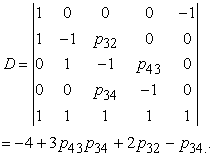AlsoTherefore, by the Cramer’s rule one finds that(7).

In the same way one finds that(8)

Furthermore, since S1 is the starting state of the EMC, with the help of equation (6) it becomes evident that the sum(9)

gives the mean number of steps of the EMC between two successive occurrences of the state S5, i.e. the mean number of steps for the completion of the process of teaching a subject matter in the classroom.

It becomes also evident that the bigger is m, the more the student difficulties in the classroom during the teaching process. Another factor of the student difficulties is the total time spent for the completion of the teaching process. However, the time is usually fixed in a formal teaching procedure in the classroom, which means that in this case m is the unique factor measuring the student difficulties.

### 4. A Classroom Application

The following application took place recently at the Graduate Technological Educational Institute of Western Greece, when the Conic Sections were taught to a group of engineering students. Five teaching hours were spent in total on the subject for teaching the circle, the ellipse, the parabola, the hyperbola and the general equation of the Conic Sections. At the end of the teaching process, where the teaching framework of our introduction based on the principles of social constructivism was followed, a number of exercises and problems analogous to those solved in the classroom were given to students on the purpose of checking at home their understanding of the subject. A week later a written test was performed in the classroom enabling the instructor to assess the student progress.

Another important thing remaining for the instructor was to evaluate the student difficulties during the teaching process, which could help him in reorganizing properly his plans for teaching the same subject in future. This was succeeded with the help of the above developed EMC representation of the teaching process in the following simple way:

The instructor noticed that the student reactions during the teaching process for Conic Sections led to total transitions of the discussion from state S3 (formalization) back to state S2 (exploration), which gives a mean number of 2 transitions per teaching hour. Therefore, since from state S2 the chain moves always to S3 (Figure 1), we have a mean number of 3 transitions from S2 to S3 per teaching hour. The instructor also counted 15 transitions from S4 (assimilation) back to S3, i.e. a mean number of 3 transitions per teaching hour. . Therefore, since from state S3 the chain moves always to state S4 (Figure 1), we have a mean number of 4 transitions from S3 to S4 per teaching hour. Consequently, we had a mean number of 3+3 = total “arrivals” to S3, 2 “departures” from S3 to S2 and 4 “departures” from S3 to S4. Therefore p32 =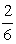and p34 =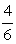. In the same way one finds that p43 =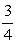and p45 =.

Replacing the above values of the transition probabilities to equation (7) one finds that p5=p1 =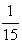. Also from equations (8) it turns out that p2 =, p3 =and P4 =. Therefore, the state of formalization (S3) is the state with the greatest gravity, which means in our case that students faced the greatest difficulties in that state of the teaching process.

Furthermore, equation (9) gives for p5=that m=14, which is equal to the mean number of steps for the completion of the teaching process in each teaching hour. Consequently, since the minimal number of steps for the completion of the teaching process is 4 (when no backward transitions exist), the students faced significant difficulties during the teaching process. Therefore the instructor should find ways to improve his teaching procedure for the same subject in future.

### 5. Conclusion

The theory of MCs, being a smart combination of Linear Algebra and Probability, offers ideal conditions for the study and mathematical modelling of a certain kind of situations depending on random variables 12, 13, 14, 15. In the paper at hands a mathematical representation of the process of teaching mathematics, based on the principles of social constructivism for learning, was developed with the help of the theory of EMCs enabling the instructor to evaluate the student difficulties during the teaching process. This is very useful for reorganizing his (her) plans for teaching the same subject in future. An application of that representation was also presented to teaching the Conic Sections to engineering students.

Although the development of the EMC model was proved to be quite laborious requiring the calculation of total determinants of fifth order, its final application is very simple. The only thing needed for this purpose is the calculation by the instructor of the transitions of the EMC from S3 back to S2 and from S4 back to S3.

Several other applications of MCs to education have been attempted by the present author in earlier works (e.g. see Chapters 2 and 3 of the book 9) and it is hoped that this research could be continued in future.

### References

  Vygotsky, L. S., Mind in society: The development of higher psychological processes. Translated by Knox and Carol, , : Press, 1978. In article  Wenger, E., Communities of Practice: Learning, Meaning, and Identity, Cambridge, Cambridge University Press, UK, 1998. In article View Article  Driver, R.; Asoko, H.; Leach, J.; Mortimer, E. & Scott, P., Constructing Scientific Knowledge in the Classroom, Educational Researcher, 23(7), 5-12, 1994. In article View Article  Voskoglou, M.Gr., Communities of Practice for Teaching and Learning Mathematics, American Journal of Educational Research, 7(6), 186-191, 2019. In article View Article  Polya G., Mathematical Discovery (2 Volumes), J.Wilet & Sons, New York, 1962/65. In article  von Glasersfeld, E., Learning as a Constructive Activity, in C. Janvier (Ed), Problems of representation in the teaching and learning of mathematics, Lawrence Erlbaum, Hillsdale, N. J., USA, pp. 3-17, 1987. In article  Polya G. , On learning, teaching and learning teaching, American. Math. Monthly, 70, 605-619, 1963. In article View Article  Kemeny, J. G. & Snell, J. L., Mathematical Models in the Social Sciences, Ginn and Company, New York, USA, 1963. In article  Voskoglou, M.Gr., Finite Markov Chain and Fuzzy Logic Assessment Models: Emerging Research and Opportunities; Createspace.com–Amazon: Columbia, SC, USA, 2017. In article  Voskoglou, M.Gr., An Absorbing Markov Chain Model for Problem Solving, American Journal of Applied Mathematics and Statistics, 4(6), 173-177, 2016. In article  Voskoglou, M.Gr., A Markov Chain Application on the Levels of the Bloom’s Taxonomy of Learning Objectives, American Journal of Educational Research, 7(3), 294-298, 2019. In article View Article  Kemeny, J. G. & Snell J. L., Finite Markov Chains, Springer - Verlag, New York, USA, 1976. In article  Domingos, P., Lowd, D., Markov Logic: An Interface Layer for Artificial Intelligence, Morgan & Claypool Publishers, Williston, VT, USA, 2009. In article View Article  Zucchini, I.L., MacDonald, I.L., Langrock, R., Hidden Markov Models for Time Series, CRC Press, NY, 2016. In article View Article  Davis, M.H.A., Markov Models and Optimization, Create Space Independent Publishing Platform, Columbia, SC., USA, 2017. In articleThis work is licensed under a Creative Commons Attribution 4.0 International License. To view a copy of this license, visit http://creativecommons.org/licenses/by/4.0/